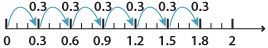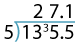Dividing decimals by decimals

When the numbers are 'nice' it is possible to perform some divisions by decimal using common sense. For example:

1.8 ÷ 0.3.

We can show what 1.8 ÷ 0.3 looks like on a number line using jumps of 0.3 until we get  to 1.8.

It takes six jumps of 0.3 to get to 0 from 1.8.Detailed description

By multiplying each number by the same multiple of ten, the division is easier to deal with.

$$1.8 ÷ 0.3 =\dfrac{1.8 × 10}{0.3 × 10}=\dfrac{18}{3}=6$$

This suggests that we multiply both numbers by the same power of 10 to make the divisor a whole number and then use the division algorithm.

1.355 ÷ 0.05 = 135.5 ÷ 5Alternatively, we can relate division of decimals to division of fractions by writing each decimal as a fraction and completing the division.

For example:

\begin{align}0.6 ÷ 0.4&=\dfrac{6}{10} ÷ \dfrac{4}{10}\\\\ &=\dfrac{6}{10} × \dfrac{10}{4}\\\\ &=\dfrac{3}{2}\\\\ &=1.5\end{align}

Example 2

Divide 0.427 by 0.07 by both methods described above.

Solution

\begin{align}0.427 ÷ 0.07&=\dfrac{0.427}{0.07}\\\\ &=\dfrac{0.427 × 100}{0.07 × 100}\\\\ &=\dfrac{42.7}{7}\\\\ &= 6.1\end{align}
\begin{align}0.427 ÷ 0.07 &=\dfrac{427}{1000}÷\dfrac{7}{100}\\\\ &=\dfrac{427}{1000} × \dfrac{100}{7}\\\\ &=\dfrac{61}{10}\\\\ &=6.1\end{align}

Summary

• To divide a decimal by a whole number, follow the same algorithm as for whole numbers.
• To divide a decimal by another decimal:
• Write the division as a quotient, and multiply both top and bottom by a power of 10 so as to make the denominator a whole number. Now proceed as above.
Or
• Convert each decimal to a fraction, perform the division and then convert back to a fraction.